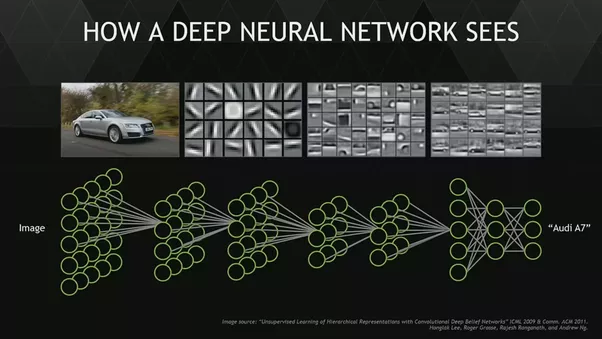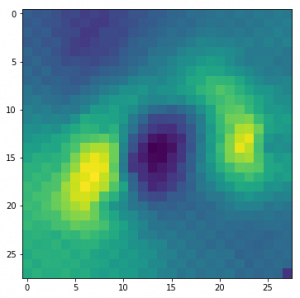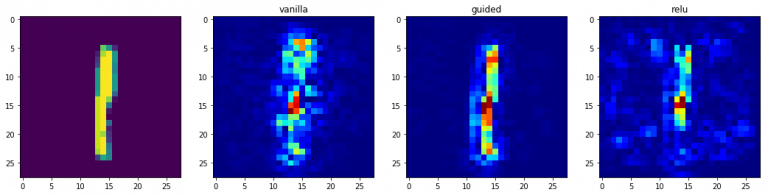# 用Python可视化卷积神经网络

### 介绍• CNN模型可视化的重要性
• 可视化方法
1. 基本方法
• 绘制模型架构
• 可视化滤波器
1. 基于激活的方法
• 最大激活
• 图像遮挡
1. 基于梯度的方法
• 显著图
• 基于梯度的类激活图

### CNN模型可视化的重要性

1. 了解模型的工作原理
2. 超参数调整
3. 找出模型的失败之处并能够解决失败
4. 向消费者/最终用户或业务主管解释决策### CNN模型的可视化方法

• 基本方法-向我们展示训练模型的总体架构的简单方法
• 基于激活的方法-在这些方法中，我们破译单个神经元或一组神经元的激活函数，以理解它们正在做什么
• 基于梯度的方法-这些方法倾向于在训练模型时操纵由向前和反向传播形成的梯度

#### 1.基本方法

##### 1.1 绘制模型架构

model.summary()

_________________________________________________________________
Layer (type)                 Output Shape              Param #
=================================================================
conv2d_1 (Conv2D)            (None, 26, 26, 32)        320
_________________________________________________________________
conv2d_2 (Conv2D)            (None, 24, 24, 64)        18496
_________________________________________________________________
max_pooling2d_1 (MaxPooling2 (None, 12, 12, 64)        0
_________________________________________________________________
dropout_1 (Dropout)          (None, 12, 12, 64)        0
_________________________________________________________________
flatten_1 (Flatten)          (None, 9216)              0
_________________________________________________________________
dense_1 (Dense)              (None, 128)               1179776
_________________________________________________________________
dropout_2 (Dropout)          (None, 128)               0
_________________________________________________________________
preds (Dense)                (None, 10)                1290
=================================================================
Total params: 1,199,882
Trainable params: 1,199,882
Non-trainable params: 0##### 1.2 可视化滤波器

top_layer = model.layers
plt.imshow(top_layer.get_weights()[:, :, :, 0].squeeze(), cmap='gray')#### 2. 基于激活的方法

##### 2.1 最大激活

from vis.visualization import visualize_activation
from vis.utils import utils
from keras import activations

from matplotlib import pyplot as plt
%matplotlib inline
plt.rcParams['figure.figsize'] = (18, 6)

# 按名称搜索图层索引。
# 或者，我们可以将其指定为-1，因为它对应于最后一层。
layer_idx = utils.find_layer_idx(model, 'preds')

#用线性层替换softmax
model.layers[layer_idx].activation = activations.linear
model = utils.apply_modifications(model)

# 这是我们要最大化的输出节点。
filter_idx = 0
img = visualize_activation(model, layer_idx, filter_indices=filter_idx)
plt.imshow(img[..., 0])PS：运行下面的脚本来检查它。

for output_idx in np.arange(10):
# 让我们这次关闭详细输出以避免混乱
img = visualize_activation(model, layer_idx, filter_indices=output_idx, input_range=(0., 1.))
plt.figure()
plt.title('Networks perception of {}'.format(output_idx))
plt.imshow(img[..., 0])

##### 2.2 图像遮挡def iter_occlusion(image, size=8):

occlusion = np.full((size * 5, size * 5, 1), [0.5], np.float32)
occlusion_center = np.full((size, size, 1), [0.5], np.float32)

), 'constant', constant_values = 0.0)

= occlusion

tmp[y:y + occlusion_center.shape, x:x + occlusion_center.shape] = occlusion_center

i = 23 # 例如
data = val_x[i]
correct_class = np.argmax(val_y[i])

# model.predict的输入向量
inp = data.reshape(1, 28, 28, 1)

# matplotlib imshow函数的图片
img = data.reshape(28, 28)

# 遮盖
img_size = img.shape
occlusion_size = 4

print('occluding...')

heatmap = np.zeros((img_size, img_size), np.float32)
class_pixels = np.zeros((img_size, img_size), np.int16)

from collections import defaultdict
counters = defaultdict(int)

for n, (x, y, img_float) in enumerate(iter_occlusion(data, size=occlusion_size)):

X = img_float.reshape(1, 28, 28, 1)
out = model.predict(X)
#print('#{}: {} @ {} (correct class: {})'.format(n, np.argmax(out), np.amax(out), out[correct_class]))
#print('x {} - {} | y {} - {}'.format(x, x + occlusion_size, y, y + occlusion_size))

heatmap[y:y + occlusion_size, x:x + occlusion_size] = out[correct_class]
class_pixels[y:y + occlusion_size, x:x + occlusion_size] = np.argmax(out)
counters[np.argmax(out)] += 1#### 3. 基于梯度的方法

##### 3.1 显著性图

class_idx = 0
indices = np.where(val_y[:, class_idx] == 1.)

# 从这里选取一些随机输入。
idx = indices

# 让sanity检查选中的图像。
from matplotlib import pyplot as plt
%matplotlib inline
plt.rcParams['figure.figsize'] = (18, 6)

plt.imshow(val_x[idx][..., 0])

from vis.visualization import visualize_saliency
from vis.utils import utils
from keras import activations

# 按名称搜索图层索引
# 或者，我们可以将其指定为-1，因为它对应于最后一层。
layer_idx = utils.find_layer_idx(model, 'preds')

# 用线性层替换softmax
model.layers[layer_idx].activation = activations.linear
model = utils.apply_modifications(model)

grads = visualize_saliency(model, layer_idx, filter_indices=class_idx, seed_input=val_x[idx])
# 可视化为热图。

# 这对应于线性层。
for class_idx in np.arange(10):
indices = np.where(val_y[:, class_idx] == 1.)
idx = indices

f, ax = plt.subplots(1, 4)
ax.imshow(val_x[idx][..., 0])

for i, modifier in enumerate([None, 'guided', 'relu']):
seed_input=val_x[idx], backprop_modifier=modifier)
if modifier is None:
modifier = 'vanilla'
ax[i+1].set_title(modifier)##### 3.2 基于梯度的类激活图

from vis.visualization import visualize_cam

# 这对应于线性层。
for class_idx in np.arange(10):
indices = np.where(val_y[:, class_idx] == 1.)
idx = indices

f, ax = plt.subplots(1, 4)
ax.imshow(val_x[idx][..., 0])

for i, modifier in enumerate([None, 'guided', 'relu']):
seed_input=val_x[idx], backprop_modifier=modifier)
if modifier is None:
modifier = 'vanilla'
ax[i+1].set_title(modifier)